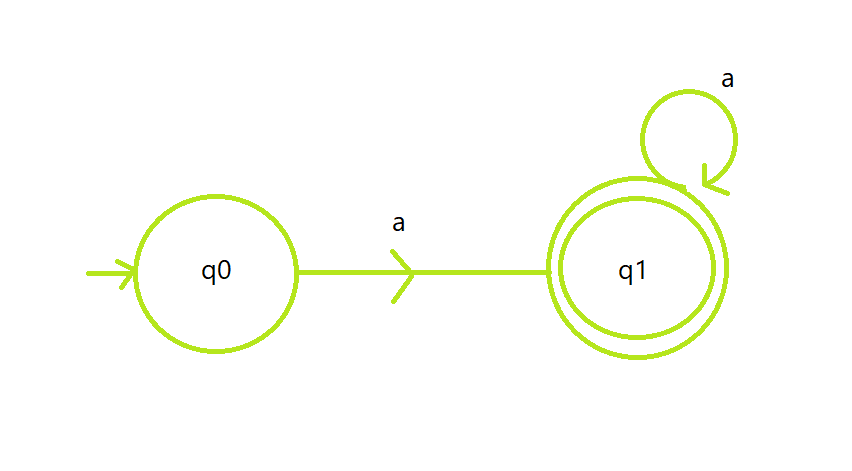Related Articles

# Program to construct a DFA which accepts the language L = {aN | N ≥ 1}

• Last Updated : 28 Jun, 2021

Prerequisite: Finite Automata

Given a string S of size N, the task is to design a Deterministic Finite Automata (DFA) for accepting the language L = {aN | N ≥ 1}. The regular language L is {a, aa, aaa, aaaaaaa…, }. If the given string follows the given language L, then print “Accepted”. Otherwise, print “Not Accepted”.

Examples:

Input: S = “aaabbb”
Output: Not Accepted
Explanation: String must only contain a.

Input: S = “aa”
Output: Accepted

Approach: The idea by which the automata lead to acceptance of string is stated below in steps:

• The automata will accept all the strings containing only the character ‘a’. If the user tried to input any character other than ‘a’, the machine will reject it.
• Let the state q0 is the initial state represent the set of all strings of length 0, state q1 is the final state represent the set of all strings from 1 to N.
• State q1 contains a self-loop of a which indicates that it can be repeated as required.
• The logic for code is very basic as it has only a for loop which counts the number of a’s in a given string, if the count of a is the same as N then it will be accepted. Otherwise, the string will be rejected.

DFA State Transition Diagram:Below is the implementation of the above approach:

## C++

 `// C++ program for the above approach` `#include ``using` `namespace` `std;` `// Function to check whether the string``// S satisfy the given DFA or not``void` `isAcceptedDFA(string s, ``int` `N)``{``    ``// Stores the count of characters``    ``int` `count = 0;` `    ``// Iterate over the range [0, N]``    ``for` `(``int` `i = 0; i < N; i++) {` `        ``// Count and check every``        ``// element for 'a'``        ``if` `(s[i] == ``'a'``)``            ``count++;``    ``}` `    ``// If string matches with DFA``    ``if` `(count == N && count != 0) {``        ``cout << ``"Accepted"``;``    ``}` `    ``// If not matches``    ``else` `{``        ``cout << ``"Not Accepted"``;``    ``}``}` `// Driver Code``int` `main()``{``    ``string S = ``"aaaaa"``;` `    ``// Function Call``    ``isAcceptedDFA(S, S.size());` `    ``return` `0;``}`

## Java

 `// Java program for the above approach``class` `GFG``{` `// Function to check whether the String``// S satisfy the given DFA or not``static` `void` `isAcceptedDFA(String s, ``int` `N)``{``    ``// Stores the count of characters``    ``int` `count = ``0``;` `    ``// Iterate over the range [0, N]``    ``for` `(``int` `i = ``0``; i < N; i++)``    ``{` `        ``// Count and check every``        ``// element for 'a'``        ``if` `(s.charAt(i) == ``'a'``)``            ``count++;``    ``}` `    ``// If String matches with DFA``    ``if` `(count == N && count != ``0``)``    ``{``        ``System.out.print(``"Accepted"``);``    ``}` `    ``// If not matches``    ``else``    ``{``        ``System.out.print(``"Not Accepted"``);``    ``}``}` `// Driver Code``public` `static` `void` `main(String[] args)``{``    ``String S = ``"aaaaa"``;` `    ``// Function Call``    ``isAcceptedDFA(S, S.length());``}``}` `// This code is contributed by 29AjayKumar`

## Python3

 `# Python3 program for the above approach` `# Function to check whether the string``# S satisfy the given DFA or not``def` `isAcceptedDFA(s, N):``  ` `    ``# Stores the count of characters``    ``count ``=` `0` `    ``# Iterate over the range [0, N]``    ``for` `i ``in` `range``(N):` `        ``# Count and check every``        ``# element for 'a'``        ``if` `(s[i] ``=``=` `'a'``):``            ``count ``+``=` `1` `    ``# If string matches with DFA``    ``if` `(count ``=``=` `N ``and` `count !``=` `0``):``        ``print` `(``"Accepted"``)` `    ``# If not matches``    ``else` `:``        ``print` `(``"Not Accepted"``)` `# Driver Code``if` `__name__ ``=``=` `'__main__'``:``    ``S ``=` `"aaaaa"` `    ``# Function Call``    ``isAcceptedDFA(S, ``len``(S))` `# This code is contributed by mohit kumar 29`

## C#

 `// C# program for the above approach``using` `System;``class` `GFG``{` `// Function to check whether the String``// S satisfy the given DFA or not``static` `void` `isAcceptedDFA(String s, ``int` `N)``{``  ` `    ``// Stores the count of characters``    ``int` `count = 0;` `    ``// Iterate over the range [0, N]``    ``for` `(``int` `i = 0; i < N; i++)``    ``{` `        ``// Count and check every``        ``// element for 'a'``        ``if` `(s[i] == ``'a'``)``            ``count++;``    ``}` `    ``// If String matches with DFA``    ``if` `(count == N && count != 0)``    ``{``        ``Console.Write(``"Accepted"``);``    ``}` `    ``// If not matches``    ``else``    ``{``        ``Console.Write(``"Not Accepted"``);``    ``}``}` `// Driver Code``public` `static` `void` `Main(String[] args)``{``    ``String S = ``"aaaaa"``;` `    ``// Function Call``    ``isAcceptedDFA(S, S.Length);``}``}` `// This code is contributed by 29AjayKumar`

## Javascript

 ``
Output:
`Accepted`

Time Complexity: O(N)
Auxiliary Space: O(1)

Attention reader! Don’t stop learning now. Get hold of all the important DSA concepts with the DSA Self Paced Course at a student-friendly price and become industry ready.  To complete your preparation from learning a language to DS Algo and many more,  please refer Complete Interview Preparation Course.

In case you wish to attend live classes with experts, please refer DSA Live Classes for Working Professionals and Competitive Programming Live for Students.

My Personal Notes arrow_drop_up